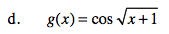### Home > CALC > Chapter 5 > Lesson 5.5.1 > Problem5-156

5-156.
1. Find the derivative of each of the following functions: Homework Help ✎

1. f(x) = x2 tan(x)

2.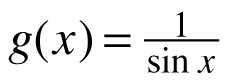3. f(x) = (2x + l)(3x − l)3

4.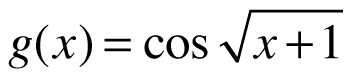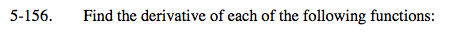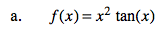Use the Product Rule.

f '(x) = 2x tan x + x2 sec2 x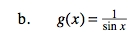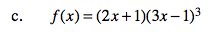Combine the Product Rule with the Chain Rule.